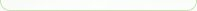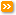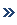Custom Search @import url(http://www.google.com/cse/api/branding.css);
 HOMEARTICLESASK AN EXPERTNEWSLETTERLIBRARYGiftednessIQ & Creativity TestAsk a QuestionMeet the ExpertGifted Child BooksIQ Testing Books# Definition of a few Terms in Educational Testing

By Inderbir Kaur Sandhu, Ph.D

Q: What do the following Educational Testing Terms mean:

NCE
Critical Value
Expected Difference
Base Rate

How are they determined?
How to interpret them?
Is Age Equivalents more reliable than Grade Equivalents?

Thank You!

A: I will try to explain the terms above as simply as possible, but it can get quite technical.

Normal curve equivalent (NCE) is a score based on the percentile rank. It indicates the position a student falls on a normal curve (a symmetrical curve/bell curve representing the normal distribution). This enables us to determine a student's rank compared to other students on the same test. Because the percentile rank scale is not an equal-interval scale (scale in which different scores represent an ordering, for e.g., from highest to lowest, and in which all scores along the scale represent the same interval). This means the difference between any two scores is not the same between any other two scores or The difference between two adjacent scores has the same meaning across the scale. This feature makes NCEs useful for comparisons between different tests. In short, NCEs are equal-interval scale conversions of percentile ranks. In educational testing, students who progress in the grade levels for example, will have a net gain in the NCE score (which means they have made progress in comparison to the general population) those while whose who show less progress would indicate a net loss in their NCE ranks.

Critical Value is used in significance testing. Significance determines if an observed value of a statistic differs enough from a hypothesized value (null hypothesis) of a parameter to draw the inference that the hypothesized value of the parameter is not the true value. It is the value that a test statistic must exceed in order for the null hypothesis to be rejected.

Expected Difference is any difference based on the average (mean) that is expected from two groups. For example, on an achievement test score, the results indicate that there was no expected difference in mean achievement test scores between group 1 (say, a group that was treated/taught with special learning methods) and for another group that was not treated. The difference is usually based on a significance level of 0.05.

Base Rate is the proportion of students in the population under study who exhibit characteristics being measured by the test. For example in an ability test, the level of criterion performance necessary for someone to be considered successful is determined. Hence, the proportion of all test-takers who would be considered successful is called the base rate.

Grade Equivalent scores determine performance in terms of theoretical level of education. It shows a child's actual performance on a test that is the number answered correctly (raw score) can be converted to a Grade Equivalent score. The Grade Equivalent score expresses the grade level of students who on average get that raw score. So, for example, if a 3rd grade child who is tested achieves a raw score of 10 points, and children near the end of 1st grade (say, at the 9th month) on average earn a raw score of 10 points, the 3rd grade child will be assigned a Grade Equivalent score of 1-9. Grade Equivalent scores are based on the assumption that it is helpful to define progress in terms of the grade-level at which an average student attains a given level of knowledge or skill. (www.ets.org/letstalk, a very interesting read for parents on testing). Grade Equivalent scores are typically only used in primary and secondary education.

Age Equivalent scores shows the typical age of the norm group that obtained a similar score. Similar to the Grade Equivalent Scores, Age Equivalent Scores allows for comparison of the childs scores with those of others who were tested on the same test. Age Equivalent Scores have the same limitations as Grade Equivalent Scores. The reliability both age and grade equivalent scores is limited by the relationship between the equivalents and the raw scores on which they are based. An age or grade equivalent is simply the median raw score for a particular age or grade level. For example, a test that measures vocabulary generally occurs more during elementary years. Therefore, the raw scores increase at a greater rate with younger examinees than with older examinees. Therefore, a similar change in raw scores of younger examinees and of older examinees will be represented quite differently in age equivalent scores. This causes the reliability for age-equivalent scores much poorer for advanced test-takers.

Hopefully the above is helpful.Checkout these other IQ questionsDecline or Increase in IQ scoresIncrease children IQ levelIQ and creativity testingIntelligence test scores classificationIntelligence (IQ) and schoolingGiftedness and reliability of IQ test scoresBack to Ask an Expert - IQ Test
 Copyright ©2002-2022 by Brainy-Child.com. Hosted by BlueHost. Privacy Statement :: Disclaimer :: Bookmark Us :: Contact Us